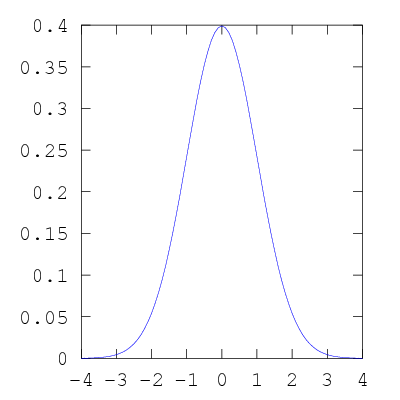# Normal probability distribution in Octave

This Octave program creates a plot of the normal probability distribution.

```x=[-4:0.1:4];
plot (x,normpdf(x,0,1));
print -dpng "-S400,400" normal.png
```